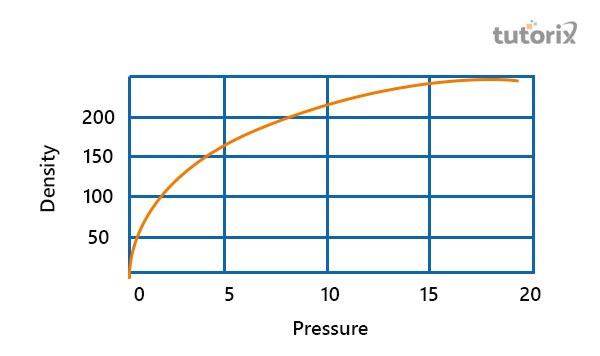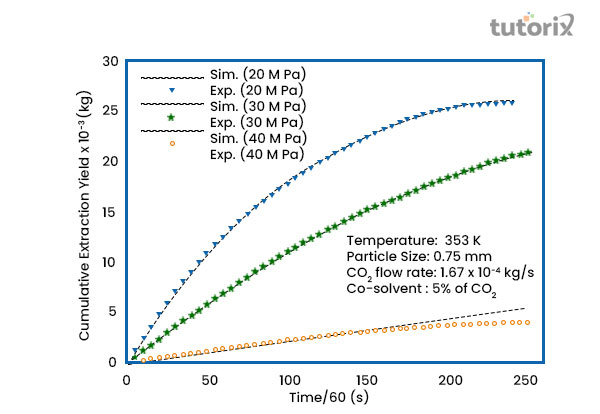# Relation Between Density and Temperature

## Introduction

A proportional relation is found within the temperature and density of an object. As a result of this relation, the changes in the surrounding temperature impact the density of an object.

The vibration within particles of an object has created as changes occur within an object. An increase in temperature impacts the overall temperature level. Vibration causes to change in the position of atoms that created heat and as a result, temperature develops.

A high temperature is integrally connected to the lower density of an object. Volumes of an object or a solution are also found to lower as the temperature decreases.

## Impacts of temperature on density

Some of the objects are found to expand after being heated. Such an example is water that expands by providing heat. The volume is also found to increase. After removing ice from the freezer if it is kept in a bowl, after some time, the ice trunks into the water, and the overall amount of volume increases.Figure 1: Relation between density and temperature

There is a direct connection between pressure and density of an object as well. The pressure increases from 0 to 5 and then to 10, the density also increases from 50, to 100 and then to 150. This states that pressure creates to increase the temperature and finally impacts increasing the density of that particular object.

## Laws related to temperature and density

Charles law is an important law with which the relation between temperature and volume of an object is examined.

In Charles Law, at the same and constant pressure, the volume and temperature of a particular gas are kept (Jia et al. 2019). This examination states that an object's volume is directly and integrally connected at a proportional level. As per the opinion of Boyle, if the volume and pressure of an object need to remain the same where the temperature is constant.

## Features of density

The temperature of an object varies with the changes in the density of this object. The mass of a particular object is eventually compared to the objected volume. The equation of density is important to understand the relation of temperate with this component (Bertini et al. 2021).

The formula is Density = mass/volume

This formula can also be presented as D = m/v. The size of the mass plays an important role in changing individual density. In different temperatures, the atoms and arrangements of an object are found to change accordingly.

## Units of temperature and density

There are four main units with which the density of an object is presented. Kg/L (Kilogram per litre) is the most used and acknowledged unit with which the density of an object is presented. G/mL (Gram per millilitre) and t/m3 (Tonne per cubic meter) are the other two important units of presenting values of densities. There are two different types of density namely relative density and absolute density (Fable et al. 2019). The changes in temperature impact both of these types of density.

The ratio between the density of reference material and the core material is regarded as the relative density (Milot et al. 2018). Most of the time, water is considered the reference material. There is another term for relative density, specific gravity.

## Features of temperature

Lattices are important components based on temperature changes.

Different kinds of wavelengths create changes in temperature.

Heat capacity changes as the components and density of an object change from time to time (Drake, 2018).

Fermi energy is the difference between the highest and the lowest temperature. This is a concept of quantum mechanics with which the differences between quantum systems are explained.

## Units of temperature

There are main three units with which temperature can be explained and these are Fahrenheit, Kelvin, and Celsius. Kelvin is the thermodynamic temperature's SI unit.Figure 2: Changes in particles with temperature

Demonstration of the relationship between

Ideal Gas Formula Symbols SI Units Full form of the terms
P = ρRT P Pa (Pascal) The pressure
R K/ J/ Mole (R=8.31) Universal constant of gas
ρ m3/Kg The density of the selected ideal gas
T K (Kelvin) The temperature of the selected gas

Table 1: Description of the relation between temperature and density in an ideal gas

## Relation between density and temperature

• In a very dense solution, the temperature is low. As the density of a solution increases in a situation, the entire temperature is found to deteriorate accordingly.
• In the opposite situation, in which the density of a solution or component decreases, the temperature increases.
• At a certain reduction of density, the density is reduced immediately (Agterberg et al. 2019).
• In relation to thermodynamics, these changes impact the overall condition and features of a solution and component.

## Conclusion

Temperature is generally the state of hotness and coldness of an individual or particle's body. Changes in places of molecules and particles play an important role in determining the changes in temperature. The knowledge about the relationship of density with temperature is important to maintaining a plumbing system, in the management and driving process of ships and submarines, the integral relation of temperature and density is required. In the time of a highly tempted molecule and object, the decrease of density can help in reducing the overall temperature.

## FAQs

Q1. What are the three different units with which various values of densities are presented?

The density of solids is presented by g/cm3. The densities of liquids and gases are presented by stating g/ml and g/L.

Q2. What is the reason behind impacting temperature by changing density?

Changes in density create changes within the place of molecules and finally affect the temperature. In the case of creating more space as well, these changes are generally done.

Q3. Are changes in temperature also effective for liquid objects?

The volume of liquids is often increased by an increase in temperature. As a result, the included molecules are also increased. This is the result of changes in temperate for a liquid.

Q4. How do changes in an object's temperature in an air medium affect its density?

The lower temperature often leads an object to have a high temperature, even in the air medium. The volume and density impact the changes in gravity. As a result, the temperature of every different place varies.

## References

### Journals

Agterberg, D. F., Davis, J. C., Edkins, S. D., Fradkin, E., Van Harlingen, D. J., Kivelson, S. A., ... & Wang, Y. (2019). The physics of pair density waves. arXiv preprint arXiv:1904.09687. Retrieved from: https://arxiv.org

Bertini, B., Heidrich-Meisner, F., Karrasch, C., Prosen, T., Steinigeweg, R., & Žnidarič, M. (2021). Finite-temperature transport in one-dimensional quantum lattice models. Reviews of Modern Physics, 93(2), 025003. Retrieved from: https://arxiv.org

Drake, R. P. (2018). Introduction to high-energy-density physics. In High-Energy-Density Physics (pp. 1-20). Springer, Cham. Retrieved from: http://nozdr.ru

Fable, E., Angioni, C., Bobkov, V., Stober, J., Bilato, R., Conway, G. D., ... & Zohm, H. (2019). The role of the source versus the collisionality in predicting a reactor density profile as observed on ASDEX Upgrade discharges. Nuclear Fusion, 59(7), 076042. Retrieved from: https://pure.mpg.de

Fu, W. J., Pawlowski, J. M., & Rennecke, F. (2020). QCD phase structure at finite temperature and density. Physical Review D, 101(5), 054032. Retrieved from: https://link.aps.org/pdf/10.1103/PhysRevD.101.054032

Jia, X., Willard, J., Karpatne, A., Read, J., Zwart, J., Steinbach, M., & Kumar, V. (2019, May). Physics guided RNNs for modeling dynamical systems: A case study in simulating lake temperature profiles. In Proceedings of the 2019 SIAM International Conference on Data Mining (pp. 558-566). Society for Industrial and Applied Mathematics. Retrieved from: https://epubs.siam.org

Milot, R. L., Klug, M. T., Davies, C. L., Wang, Z., Kraus, H., Snaith, H. J., ... & Herz, L. M. (2018). The effects of doping density and temperature on the optoelectronic properties of formamidinium tin triiodide thin films. Advanced Materials, 30(44), 1804506. Retrieved from: https://onlinelibrary.wiley.com

Websites